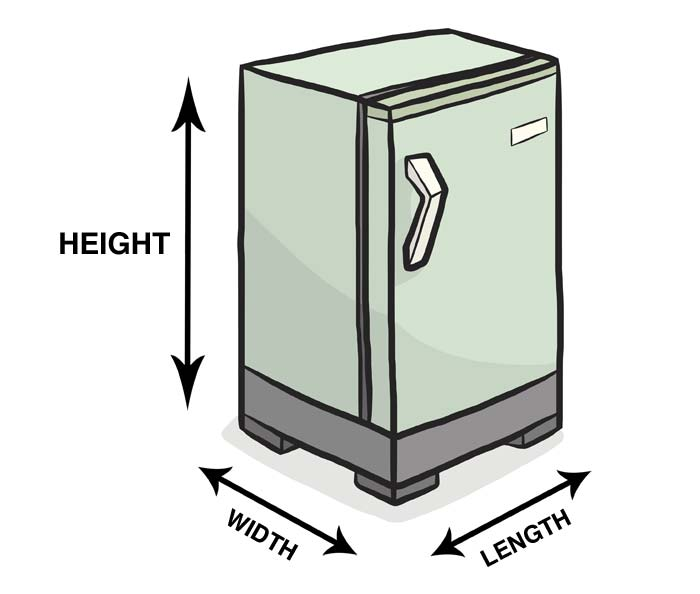# How To Calculate Cubic Feet

| Last update: 27 November 2019

It's not an everyday problem, but there will be times when you need to calculate the capacity of something. Instead of staring blankly into the trunk of your car, getting a tension headache trying to find the biggest bathtub, or weeping when confronted by the decision over buying a new fridge-freezer or oven, just take control!## How to do it

There are only three measurements you need to take to work out the volume or capacity in cubic feet: length, width and height. It's worth being aware of the object you're measuring, however. For example, if you're aiming to measure the cubic feet capacity of a refrigerator, you need to measure the space inside the fridge, and not the dimensions of the exterior. You can read instructions for measuring a refrigerator here.Once you've obtained the three measurements, it's only a case of multiplying them together. Or, if you prefer, you can let the cubic feet calculator do the hard work for you.

Length (feet) x Width (feet) x Height (feet) = Volume in cubic feet (written as Xft3)

If the shape is irregular, mentally divide it into regular shapes and calculate the volume for each part separately, before adding them all together.

For example, an L shaped cabinet designed to fit the corner of a room could be dealt with as two different rectangles, one longer, one shorter. Just work out the capacity of each one individually and then add them together for the total capacity of the cabinet.

## Converting to cubic feet from other units

If you're shopping online, the dimensions of something may only be given in inches. If you're looking at plans for a room, maybe the figures are given in metres or yards. But don't worry! To arrive at the cubic feet measurement, you only need to add one extra step into your calculations...

• If you need to convert to cubic feet from inches: divide the final number (i.e. the total you get after multiplying the three dimensions together) by 1728.
• If you need to convert to cubic feet from metres: multiply your final number by 35.31.
• If you need to convert to cubic feet from yards: multiply your final number by 27.

## Real world examples

Let's take a look at a couple of examples of situations where you may need to calculate cubic feet...

### Example 1

I want to buy a dressing-up box for my daughter, but one looks longer while the other looks taller. I can't judge by eye which one can store the most costumes.

Box A is 70" x 30" x 30" = 63,000".

63,000 divided by 1728 (cubic inches to cubic ft conversion) = 36.45. So it is 36.45ft3

Box B is 60" x 28" x 45" = 75,600".

75,600 divided by 1728 (cubic inches to cubic ft conversion) = 43.75.

So it is 43.75ft3. Box B has the larger capacity, so I will buy that one.

Let's try another...

### Example 2

My friend is driving us on a trip, and I need to work out how much stuff I can bring.

The trunk of her car is L shaped, because there's a storage section built into one corner to hold a toolbox. The largest rectangle of the L is 60" x 30" x 35" and the smaller rectangle is 18" x 14" x 35".

Larger rectangle

60 x 30 x 35 = 63,000.
63,000 divided by 1728 = 36.45.
36.45ft3

Smaller rectangle

18 x 14 x 35 = 8,820.
8,820 divided by 1728 = 5.10.
5.10ft3

And the two added together (36.45ft3 + 5.1ft3 ) makes a total capacity of 41.55ft3.

If you would still like help calculating the cubic feet volume of an item, give the cubic feet calculator a try.